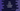# C++ program to print a random number## Random number generator in C++ :

This tutorial is to write one Random number generator program in C++. Actually it is hard to create one random number generator without using any library. You need some start point that is always different and always creates one random value. For example, time. We can take the current time as the starting point and write one function that creates one random value using it.

Luckily, C++ provides us one inbuilt library function to create random numbers easily. I will show you one example program that will create 10 random numbers each time you run it.

### rand() function :

rand() function is used to create random numbers. This is defined in stdlib.h. Not only in C++, you can also use this function in C. It returns one random value and it is in the range of 0 to RANDMAX. _RANDMAX_ is a constant and its value may differ.

### srand() :

srand or pseudo-random generator is an initializer function for rand. It takes one integer value. We should always call this function before calling rand. Else, rand will always return same set of random values. Let’s take a look at the below example :

``````#include <iostream>
#include <stdlib.h>
using namespace std;
int main()
{
for (int i = 0; i < 4; i++)
{
cout << "Random number : " << rand() << endl;
}
return 0;
}``````

If you run it, it will print the same results. We are using one loop that calls rand() four times but we are not using srand here.

That is the use of srand. If you don’t use it, rand() will always print the same value.

### Using srand and time :

srand is used to initialize the rand execution with a seed. To make it really random, i.e. to generate different random numbers each time the program executes, we need to pass different integer values to it. time(NULL) returns the number of seconds since Jan 1, 1970. So, it will be always different and we can pass it as seed to srand :

``````#include <iostream>
#include <stdlib.h>
#include <time.h>
using namespace std;
int main()
{
srand(time(NULL));
for (int i = 0; i < 4; i++)
{
cout << "Random number : " << rand() << endl;
}
return 0;
}``````

You need to include time.h to use time() function. It will always print different random number.

### Random number less than a specific number :

We can use modulo % to create random numbers less than a given number. m % n returns the remainder of m/n. For example, if you use rand()%1000, it will always return numbers less than 1000.

Try to create random numbers less than a given number and drop your code comment below :)